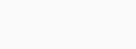# How to write DDA Line Drawing Algorithm using Java Applet

In this tutorial, we will learn how to draw a line by using DDA Line drawing algorithm. Even though many websites have the codes available but there were no codes available for the Java Applet and since this problem was handled by me in at University I thought to share my Code here at CodeSpeedy.

## DDA Line Drawing Algorithm

Let’s see how it works.

The DDA algorithm interpolates values in interval by computing for each xi the equations xi = xi−1 + 1,  yi = yi−1 + m, where m is the slope of the line. This slope can be expressed in DDA as follows:We will start by importing the necessary packages.

```package computergraphics;

import java.io.*;
import java.awt.*;
import java.awt.image.*;
import java.applet.*;
import java.util.*;
```

Then we will generate our writable raster, which will be responsible for plotting our points and showing them on screen.

```BufferedImage image = new BufferedImage(100, 150, BufferedImage.TYPE_INT_ARGB);
WritableRaster raster = image.getRaster();
int array[] = {250, 0, 0, 250};

public void init() {
setSize(800, 800);
}
```

Now our paint method will execute our full algorithm and we will be using raster.setPixel method in order to plot our points.

My Full Code for the algorithm looks like:-

```package computergraphics;

import java.io.*;
import java.awt.*;
import java.awt.image.*;
import java.applet.*;
import java.util.*;

public class Dda extends Applet {

BufferedImage image = new BufferedImage(100, 150, BufferedImage.TYPE_INT_ARGB);
WritableRaster raster = image.getRaster();
int array[] = {250, 0, 0, 250};

public void init() {
setSize(800, 800);
}

public void paint(Graphics g) {
double dx, dy, x, y;
int k;
double xc, yc;
double x1 = 20, y1 = 50, x2 = 60, y2 = 70;

dx = x2 - x1;
dy = y2 - y1;

double m = dy / dx;

if (m <= 1) {
xc = 1;
yc = m;
} else {
xc = 1 / m;
yc = 1;
}

x = x1;
y = y1;
raster.setPixel((int) Math.round(x), (int) Math.round(y), array);
System.out.println("k = 0 : " + (int) Math.round(x) + " , " + (int) Math.round(y));

for (k = 1; k <= dx; k++) {
x += xc;
y += yc;
raster.setPixel((int) Math.round(x), (int) Math.round(y), array);
System.out.println("k = " + k + " : " + (int) Math.round(x) + " , " + (int) Math.round(y));
}
g.drawImage(image, 0, 0, this);
}
}

```

The output for the code will be an applet viewer with the line drawn on the co-ordinates.

Note – In order to run this file without any issues use NetBeans and press Shift+F6 to execute the code.

### One response to “How to write DDA Line Drawing Algorithm using Java Applet”

1. ABDULRAZAK SIKIRU says:

Thank you for the Contribution
Sir,
I have type every code into my computer while running showing error message “could not find main class “MidPointCircleDrawing
But it’s successfully compiled